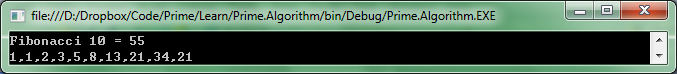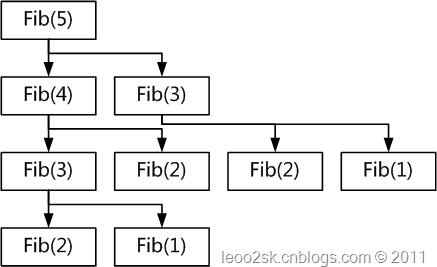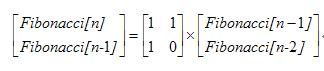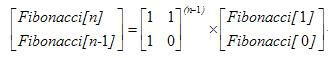# 【求职面试】斐波那契数列（C#版）

1，有一段楼梯有10级台阶,规定每一步只能跨一级或两级,要登上第10级台阶有几种不同的走法?

2，数列中相邻两项的前项比后项的极限是多少，就是问，当n趋于无穷大时，F(n)/F(n+1)的极限是多少？

Fibonacci数列的数学表达式就是：

F(n) = F(n-1) + F(n-2)

F(1) = 1

F(2) = 1

如果起点是0的话，对于第一级台阶来讲有F(1)=1种走法，对于第二级来讲有F(2)=1种走法。对于第三极台阶，有1+2, 2+1两种走法。对于第四级台阶，可以从第三极台阶+1，也可以从第二级台阶+2，也就是F(4) = F(3) + F(2)，以此类推。

写代码也很简单：

        ///<summary>        /// Fibonacci递归算法。时间复杂度O(n)=O((3/2)^n)，指数级算法        ///</summary>        public ulong FibonacciRecursion(int n)        {            if (n < 0)                throw new ArgumentOutOfRangeException("n must > 0.");            if (n == 1 || n == 2)                return 1;            return FibonacciRecursion(n - 1) + FibonacciRecursion(n - 2);        }

        ///<summary>        /// 递归输出Fibonacci        ///</summary>        public ulong FibonacciRecursionCount(int n, int[] countArray)        {            countArray[n]++;  //count the compute number.            if (n < 0)                throw new ArgumentOutOfRangeException("n must > 0.");            if (n == 1 || n == 2)                return 1;            return FibonacciRecursionCount(n - 1, countArray) + FibonacciRecursionCount(n - 2, countArray);        }fib(10) = 1     fib(9) = 1      fib(8) = 2      fib(7) = 3

fib(6) = 5      fib(5) = 8      fib(4) = 13    fib(3) = 21

fib(2) = 34   fib(1) = 55    fib(0) = 34

T(N) = T(N-1) + T(N-2) + 2

fib(N) < (5/3)^N

N>4时，fibN>= (3/2)^N///<summary>        /// 尾递归算Fibonacci。算法时间复杂度O(n) = n        ///</summary>        ///<remarks>        /// 与普通递归相比，由于尾递归的调用处于方法的最后，因此方法之前所积累下的各种状态对于递归调用结果已经没有任何意义        /// 因此完全可以把本次方法中留在堆栈中的数据完全清除，把空间让给最后的递归调用。        /// 这样的优化便使得递归不会在调用堆栈上产生堆积，意味着即时是“无限”递归也不会让堆栈溢出。（实际中，还是会溢出的！）        /// 这便是尾递归的优势。        ///         /// 尾递归的本质，其实是将递归方法中的需要的“所有状态”通过方法的参数传入下一次调用中。        ///</remarks>        public ulong FibonacciTailCall(int n, ulong sum, ulong accumulator)        {            if (n < 0)                throw new ArgumentOutOfRangeException("n must > 0.");            //n代表起点。也就是倒着算，从第n阶直到第0阶（起点）。循环n+1次。            if (n == 0)                return sum;   //sum是结果，初始值应该传0            /* 传入状态：             * 1. n每次都减一             * 2. 传入accumulator表示把上一次的累加值当做这次的Sum             * 3. 表示累加运算（如果是n!阶乘运算，就是* 乘法）            */            return FibonacciTailCall(n - 1, accumulator, sum + accumulator);         }        public void TestFibonacciTailCall()        {            //sum初始值为0；累加器为1            FibonacciTailCall(10, 0, 1);        }

        ///<summary>        /// 使用Stack来实现Fibonacci，算法时间复杂度O(n) = n        ///</summary>        public ulong FibonacciStack(int n)        {            if (n < 0)                throw new ArgumentOutOfRangeException("n must > 0.");            if (n == 1 || n == 2)                return 1;            //只需要2个元素，用2来节省内部数组占用的内存            Stack<ulong> stack = new Stack<ulong>(2);            stack.Push(1);            stack.Push(1);            //从3开始（F(3)），直到n（F(n)）            for (int i = 3; i <= n; i++)            {                var top = stack.Pop();                var bottom = stack.Pop();                var sum = top + bottom;                stack.Push(top);                stack.Push(sum);            }            return stack.Peek();        }

        ///<summary>        /// 使用Queue来实现Fibonacci，算法时间复杂度O(n) = n        ///</summary>        public ulong FibonacciQueue(int n)        {            if (n < 0)                throw new ArgumentOutOfRangeException("n must > 0.");            if (n == 1 || n == 2)                return 1;            //只需要2个元素，用2来节省内部数组占用内存            Queue<ulong> queue = new Queue<ulong>(2);            queue.Enqueue(1);            queue.Enqueue(1);            //从3开始（F(3)），直到n（F(n)）            for (int i = 3; i <= n; i++)            {                var front = queue.Dequeue();                var end = queue.Dequeue();                var sum = front + end;                //为了提高效率，采用倒序queue， 也就是 8,5,3,2,1,1                queue.Enqueue(sum);                queue.Enqueue(front);            }            return queue.Peek();        }

        ///<summary>        /// 使用数组模拟Stack来实现Fibonacci，算法时间复杂度O(n) = n        ///</summary>        public ulong FibonacciArray(int n)        {            if (n < 0)                throw new ArgumentOutOfRangeException("n must > 0.");            if (n == 1 || n == 2)                return 1;            ulong[] array = new ulong[] { 1, 1 };            //模拟Stack结构实现            for (int i = 3; i <= n; i++)            {                var sum = array + array;                array = array;                array = sum;            }            return array;        }

        public static int CalculateFibonacciSequence(int index)        {            if (index <= 0)            {                return 0;            }            if (index == 1 || index == 2)            {                return 1;            }            int[] fibonacciArray = new int[index];            fibonacciArray = 1;            fibonacciArray = 1;            for (int innerIndex = 2; innerIndex < fibonacciArray.Length; innerIndex++)            {                fibonacciArray[innerIndex] = fibonacciArray[innerIndex - 1] + fibonacciArray[innerIndex - 2];            }            return fibonacciArray[index - 1];        }

        ///<summary>        /// 使用变量模拟Stack来实现Fibonacci，算法时间复杂度O(n) = n        ///</summary>        public ulong FibonacciVariable(int n)        {            if (n < 0)                throw new ArgumentOutOfRangeException("n must > 0.");            if (n == 1 || n == 2)                return 1;            ulong first = 1, last = 1;            //模拟Stack结构实现            for (int i = 3; i <= n; i++)            {                //巧妙的，不借助中级变量sum                last = first + last;                first = last - first;            }            return last;        }

Fibonacci = 0，Fibonacci = 1

Fibonacci[n] = Fibonacci[n-1] + Fibonacci[n-2] (n >= 2)class Matrix{public:       long matr;        Matrix(const Matrix&rhs);       Matrix(long a, long b, long c, long d);       Matrix& operator=(const Matrix&);       friend Matrix operator*(const Matrix& lhs, const Matrix& rhs)       {              Matrix ret(0,0,0,0);              ret.matr = lhs.matr*rhs.matr + lhs.matr*rhs.matr;              ret.matr = lhs.matr*rhs.matr + lhs.matr*rhs.matr;              ret.matr = lhs.matr*rhs.matr + lhs.matr*rhs.matr;              ret.matr = lhs.matr*rhs.matr + lhs.matr*rhs.matr;              return ret;       }}; Matrix::Matrix(long a, long b, long c, long d){       this->matr = a;       this->matr = b;       this->matr = c;       this->matr = d;}Matrix::Matrix(const Matrix &rhs){       this->matr = rhs.matr;       this->matr = rhs.matr;       this->matr = rhs.matr;       this->matr = rhs.matr;}Matrix& Matrix::operator =(const Matrix &rhs){       this->matr = rhs.matr;       this->matr = rhs.matr;       this->matr = rhs.matr;       this->matr = rhs.matr;       return *this;} Matrix power(const Matrix& m, int n){       if (n == 1)              return m;       if (n%2 == 0)              return power(m*m, n/2);       else              return power(m*m, n/2) * m;}long fib4 (int n){       Matrix matrix0(1, 1, 1, 0);       matrix0 = power(matrix0, n-1);       return matrix0.matr;}

### 初等代数解法

• a1 = 1
• a2 = 1
• an = an − 1 + an − 2

#### [编辑]首先构建等比数列

an + αan − 1 = β(an − 1 + αan − 2)

an = (β − α)an − 1 + αβan − 2$\begin{cases} \beta-\alpha=1 \\ \alpha\beta=1 \end{cases}$$\begin{cases} \alpha=\dfrac{\sqrt{5}-1}{2} \\ \beta=\dfrac{\sqrt{5}+1}{2} \end{cases}$

#### [编辑]求出数列{an + αan − 1}\begin{align}a_{n+1} +\alpha a_{n} &=(a_2+\alpha a_1)\beta^{n-1}\\ & = (1+\alpha)\beta^{n-1}\\ & =\beta^n \\ \end{align}

#### 求数列{bn}进而得到{an}$b_{n+1}+\frac{\alpha}{\beta}b_{n}=\frac{1}{\beta}$$b_{n+1}+\lambda=-\frac{\alpha}{\beta} (b_{n}+\lambda)$，解得$\lambda=-\frac{1}{\alpha+\beta}$。 故数列$\left\{b_n+\lambda\right\}$为等比数列$b_n+\lambda=\left(-\frac{\alpha}{\beta}\right)^{n-1}\left(b_1+\lambda\right)$。而$b_1=\frac{a_1}{\beta}=\frac{1}{\beta}$， 故有$b_n+\lambda=\left(-\frac{\alpha}{\beta}\right)^{n-1}\left(\frac{1}{\beta}+\lambda\right)$$a_{n}=\frac{\sqrt{5}}{5} \cdot \left[\left(\frac{1 + \sqrt{5}}{2}\right)^{n} - \left(\frac{1 - \sqrt{5}}{2}\right)^{n}\right]$

        ///<summary>        /// 公式法。时间复杂度O(1)，但用到了计算平方根和平方运算，实际效果不一定有O(n)的好        ///</summary>        ///<param name="n"></param>        ///<returns></returns>        public ulong FibonacciFormula(int n)        {            if (n < 0)                throw new ArgumentOutOfRangeException("n must > 0.");            if (n == 1 || n == 2)                return 1;            double z = Math.Sqrt(5.0);            double x = (1 + z) / 2;            double y = (1 - z) / 2;            double result = (Math.Pow(x, n) - Math.Pow(y, n)) / z;            return (ulong)result;        }

        ///<summary>        /// 使用BigInteger模拟Stack来实现Fibonacci，算法时间复杂度O(n) = n        ///</summary>        public BigInteger FibonacciBitInteger(int n)        {            if (n < 0)                throw new ArgumentOutOfRangeException("n must > 0.");            if (n == 1 || n == 2)                return 1;            BigInteger first = 1, last = 1;            for (int i = 3; i <= n; i++)            {                last = first + last;                first = last - first;            }            return last;        }

写代码没有对与错，只有精益求精。我很享受由浅入深的过程，在写文章的时候，自己也有所收获。当然，我把时间花在写文章的时候，也就丧失了其他机会。时间对于每个人都是公平的。有的人喜欢音乐，有的人喜欢看电影，而我喜欢用更好的方法来解决问题。